# NCERT Exemplar Class 11 Physics Solutions for Chapter 14 - Oscillations

NCERT Exemplar Class 11 Physics Chapter 14 Oscillations is a very crucial study material for the students of CBSE class 11. This chapter encompasses basic concepts that are required to understand some of the topics in class CBSE class 12.

This NCERT exemplar class 11 physics Chapter 14 pdf comprises oscillations class 11 important questions, numerical on oscillations class 11, MCQ’s, Short and long answer questions, important questions from previous year question papers and sample papers.

Oscillation can be defined as the variation that is repetitive in a certain amount of time from the beginning of a point of equilibrium. Some of the different types of oscillations are simple harmonic, damped and driven oscillation, coupled oscillations and waves.

## Download the PDF of NCERT Exemplar Class 11 Physics Chapter 14 Oscillations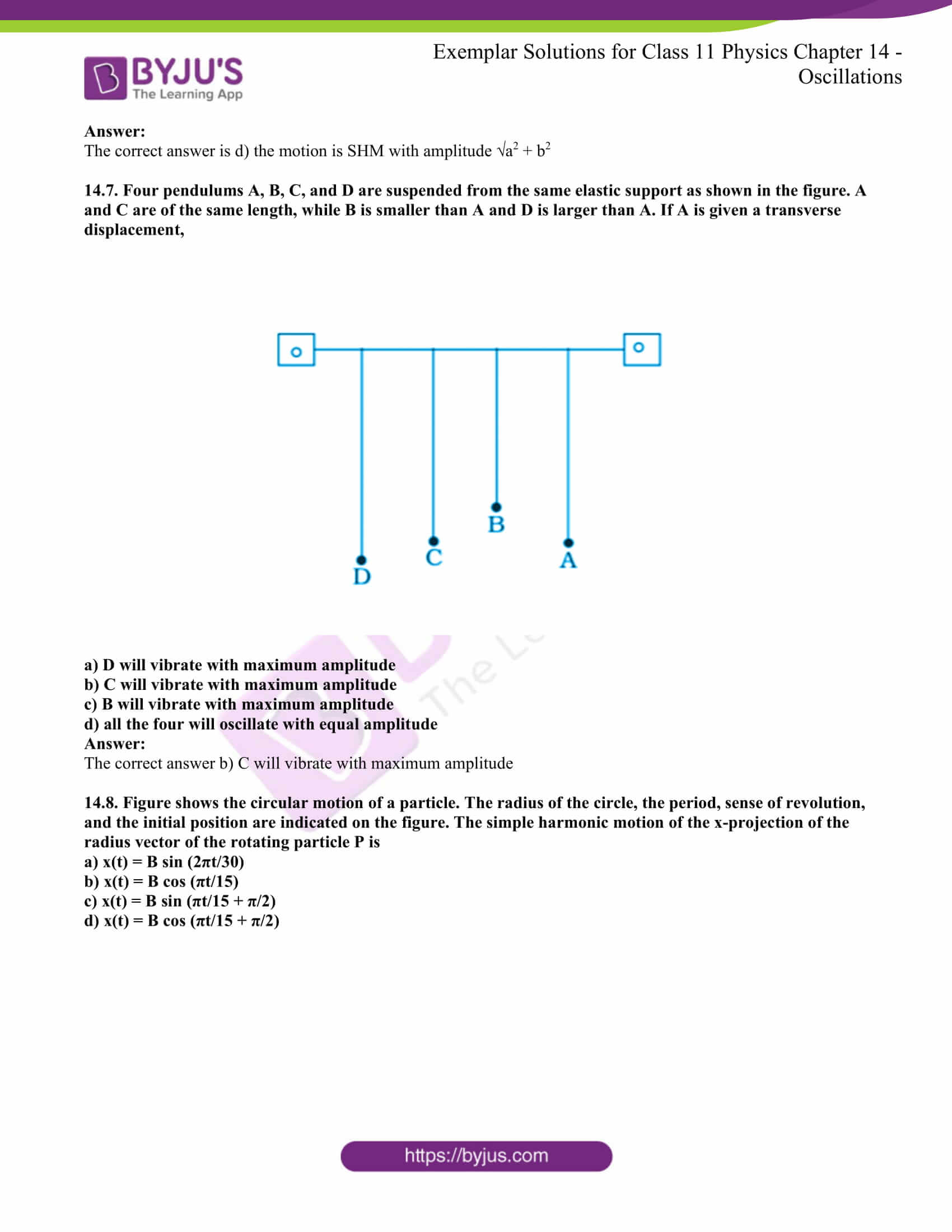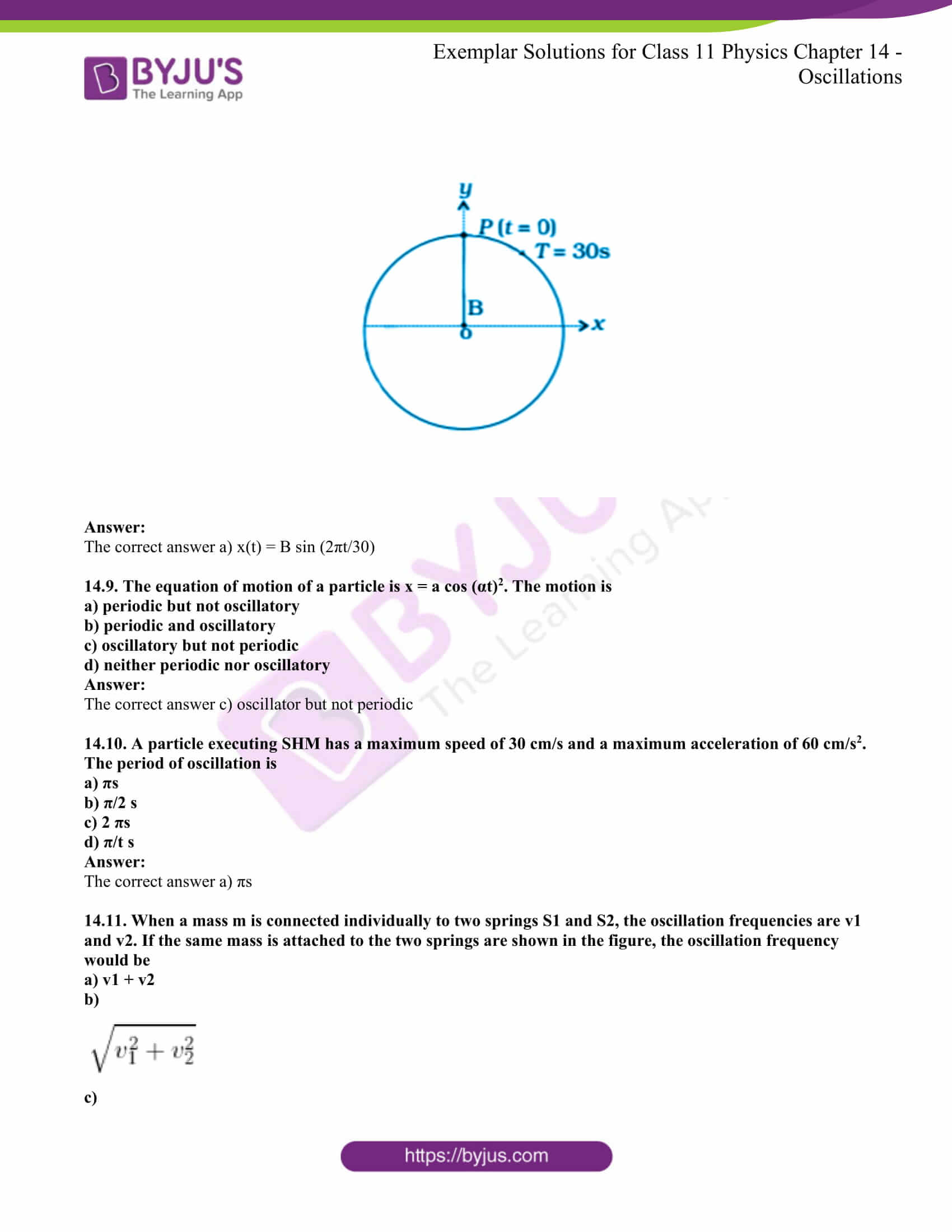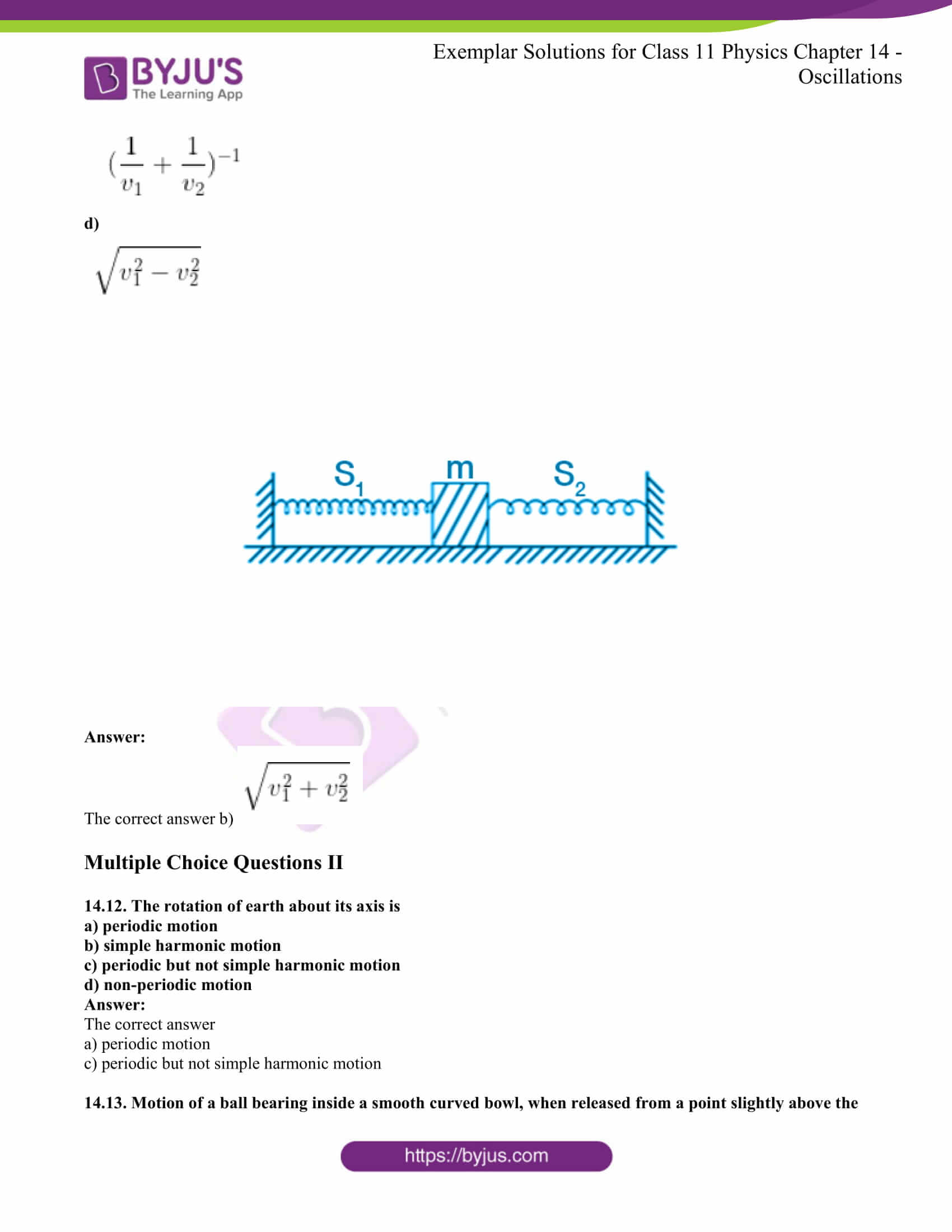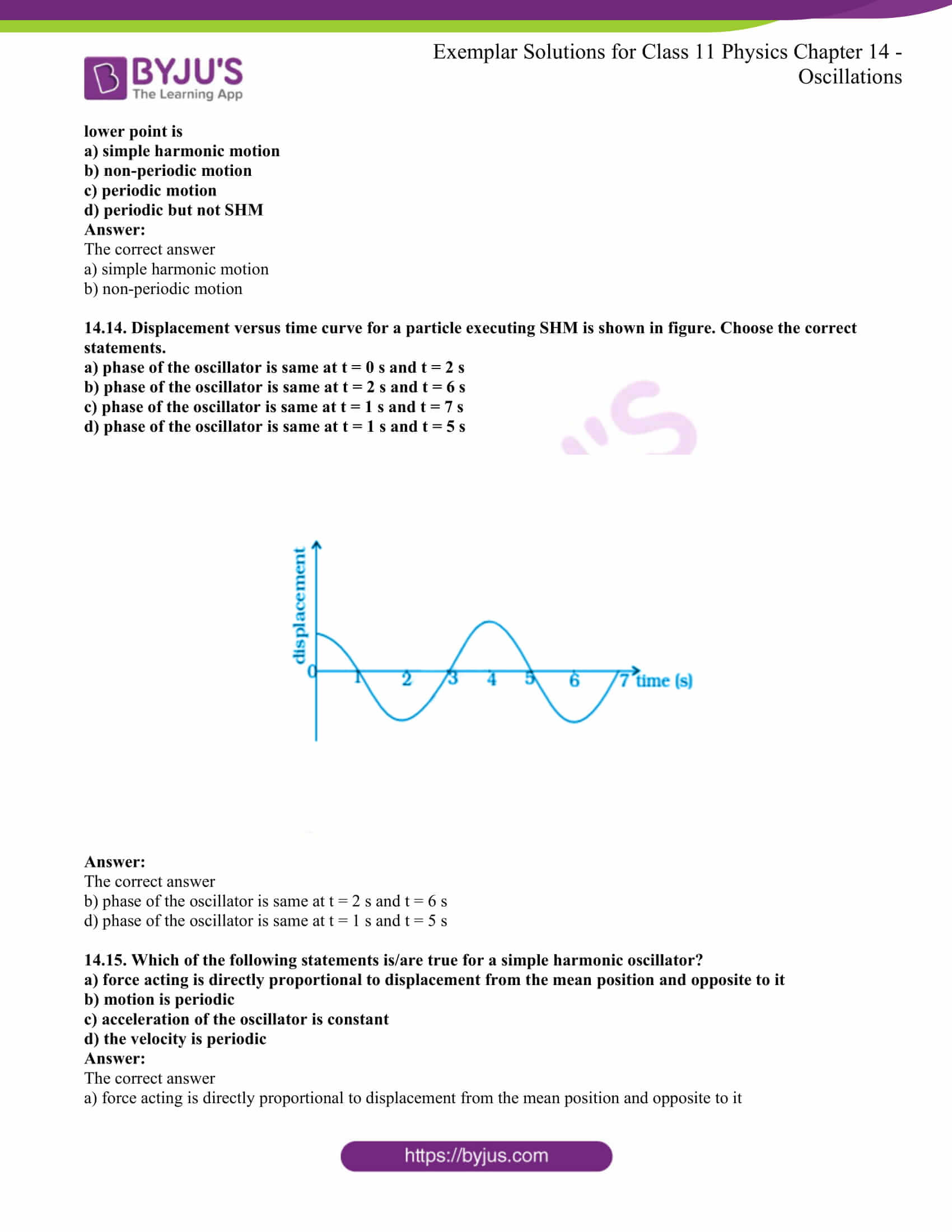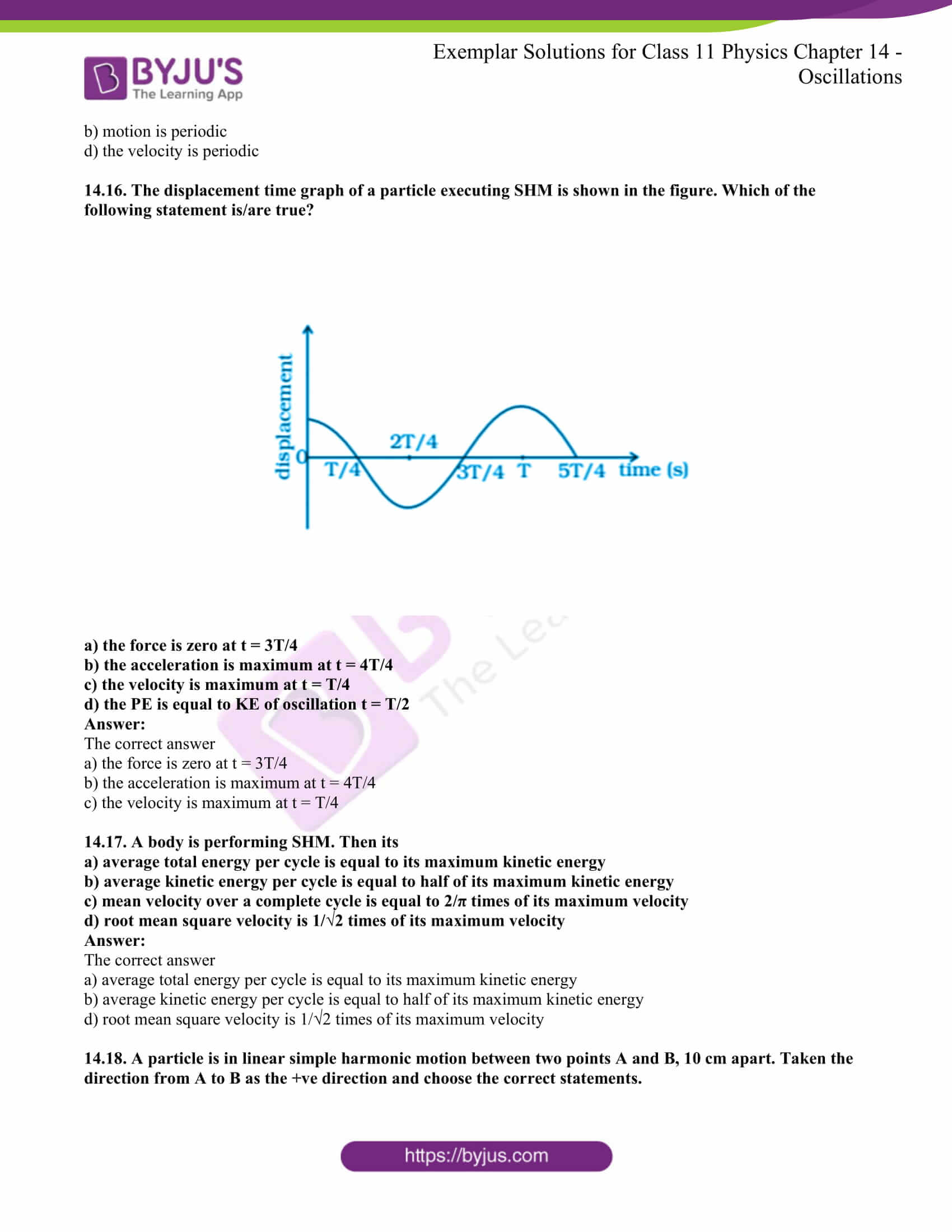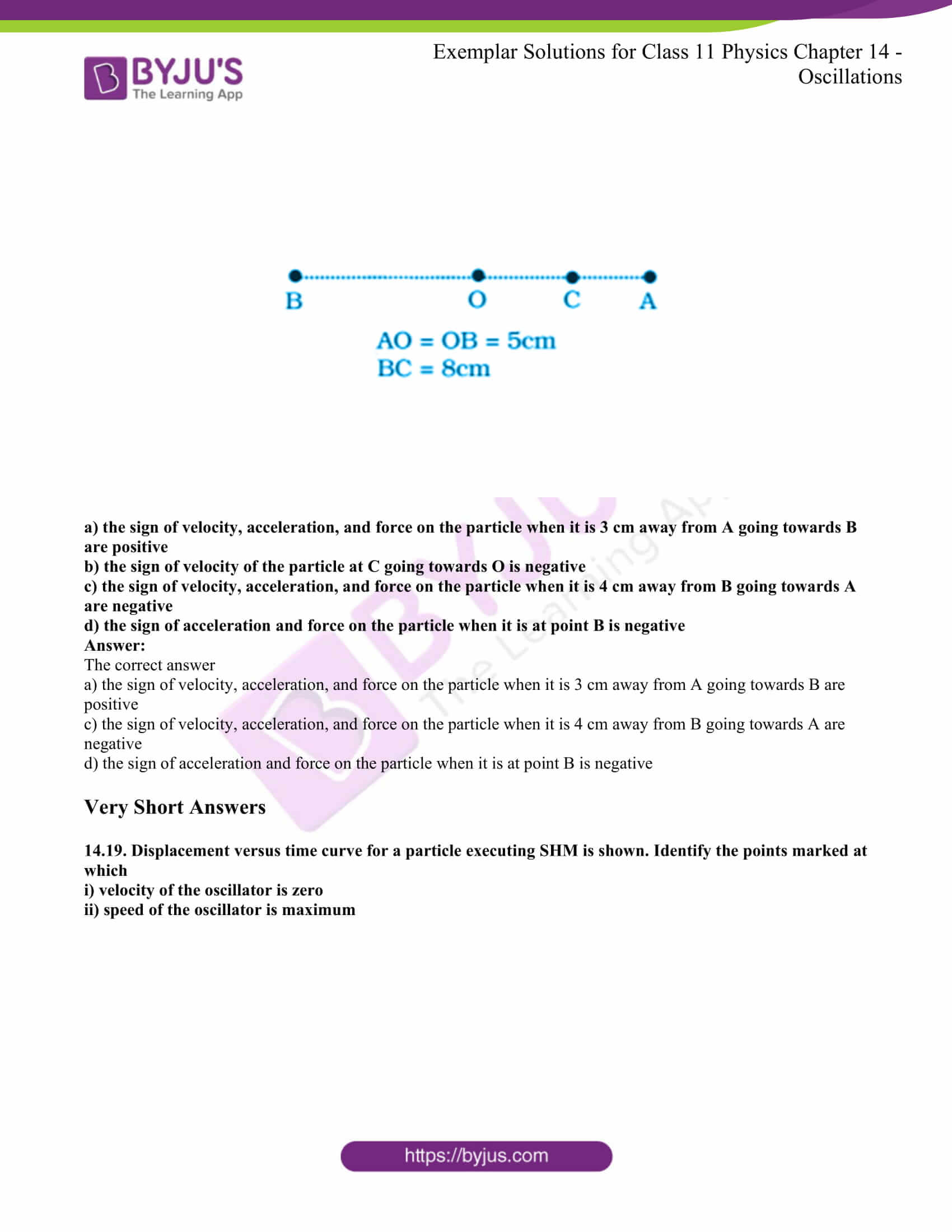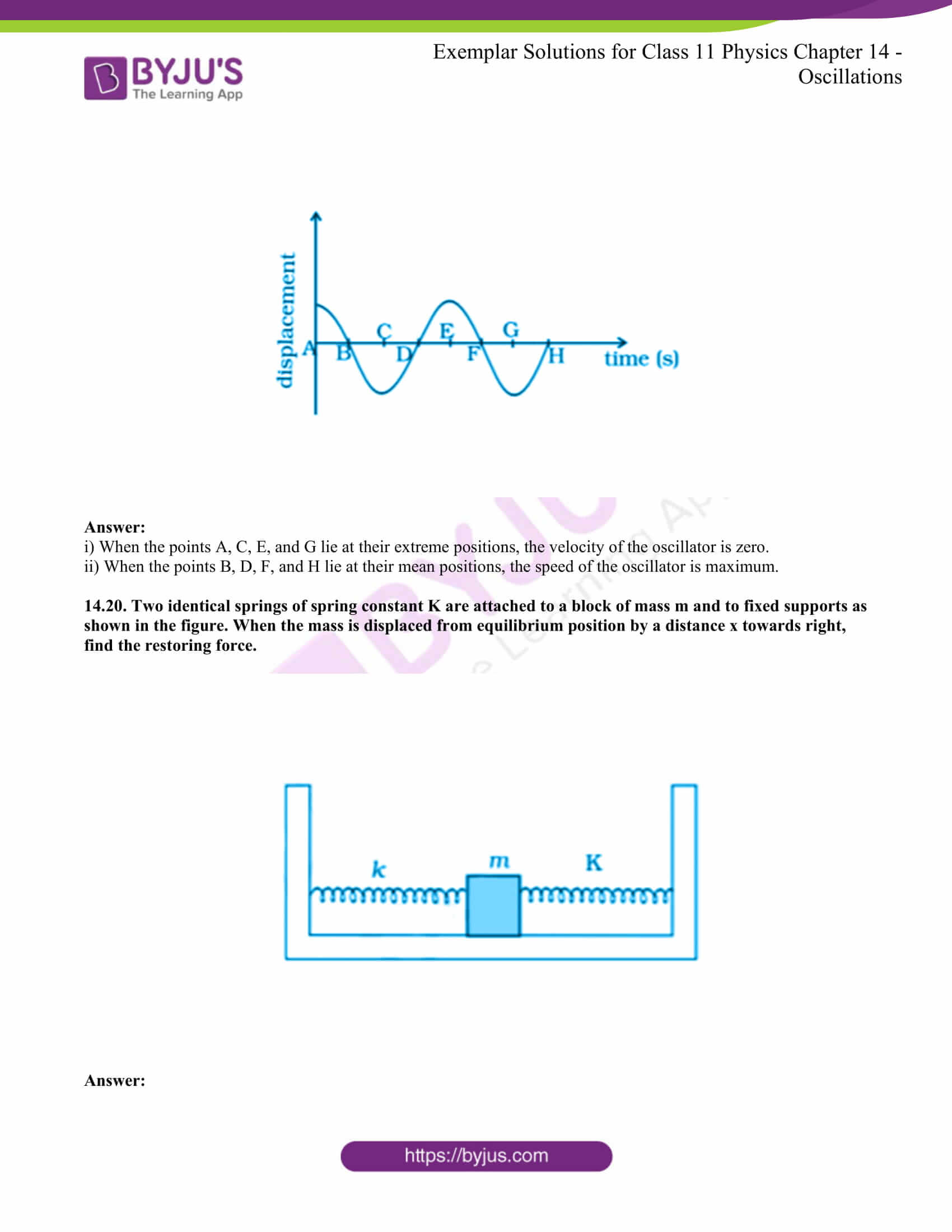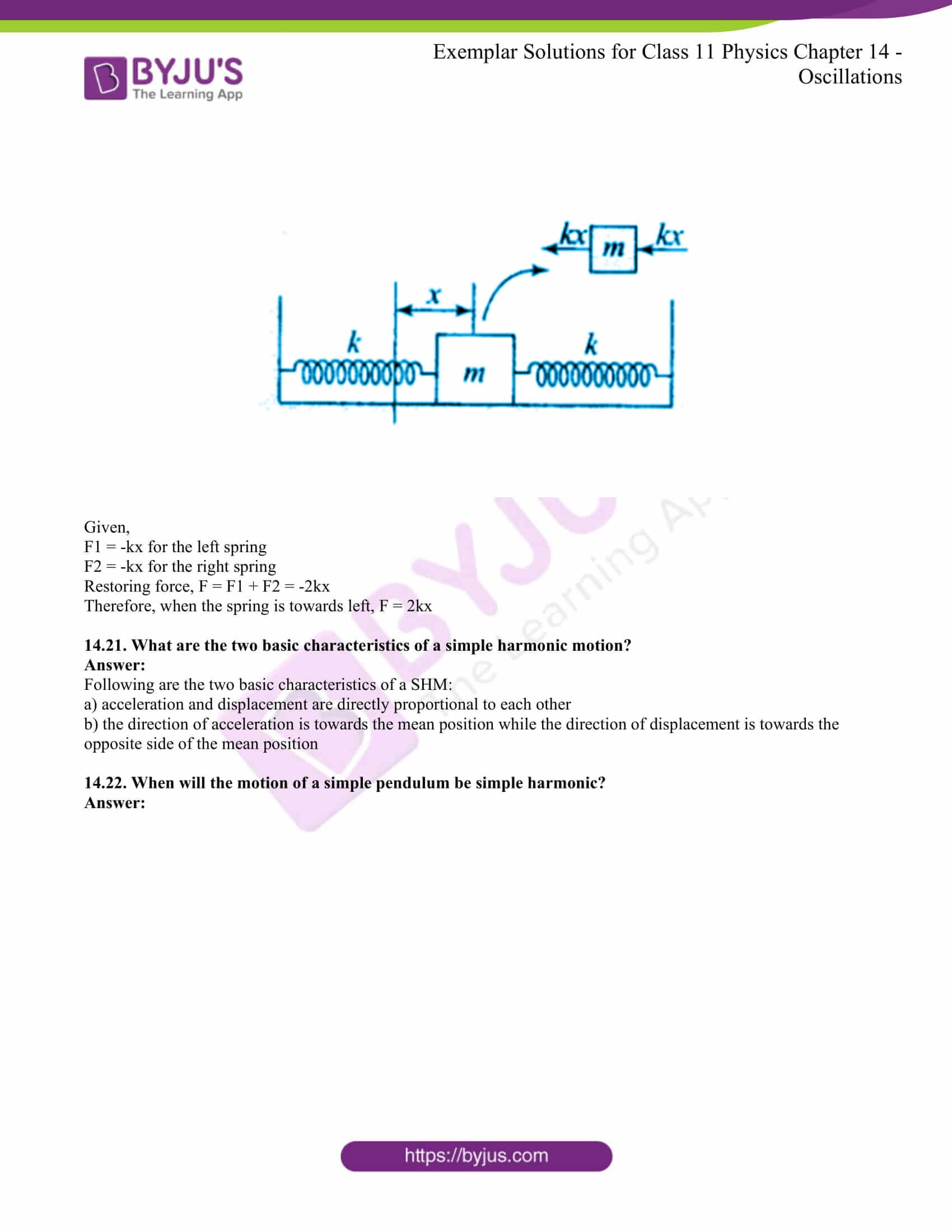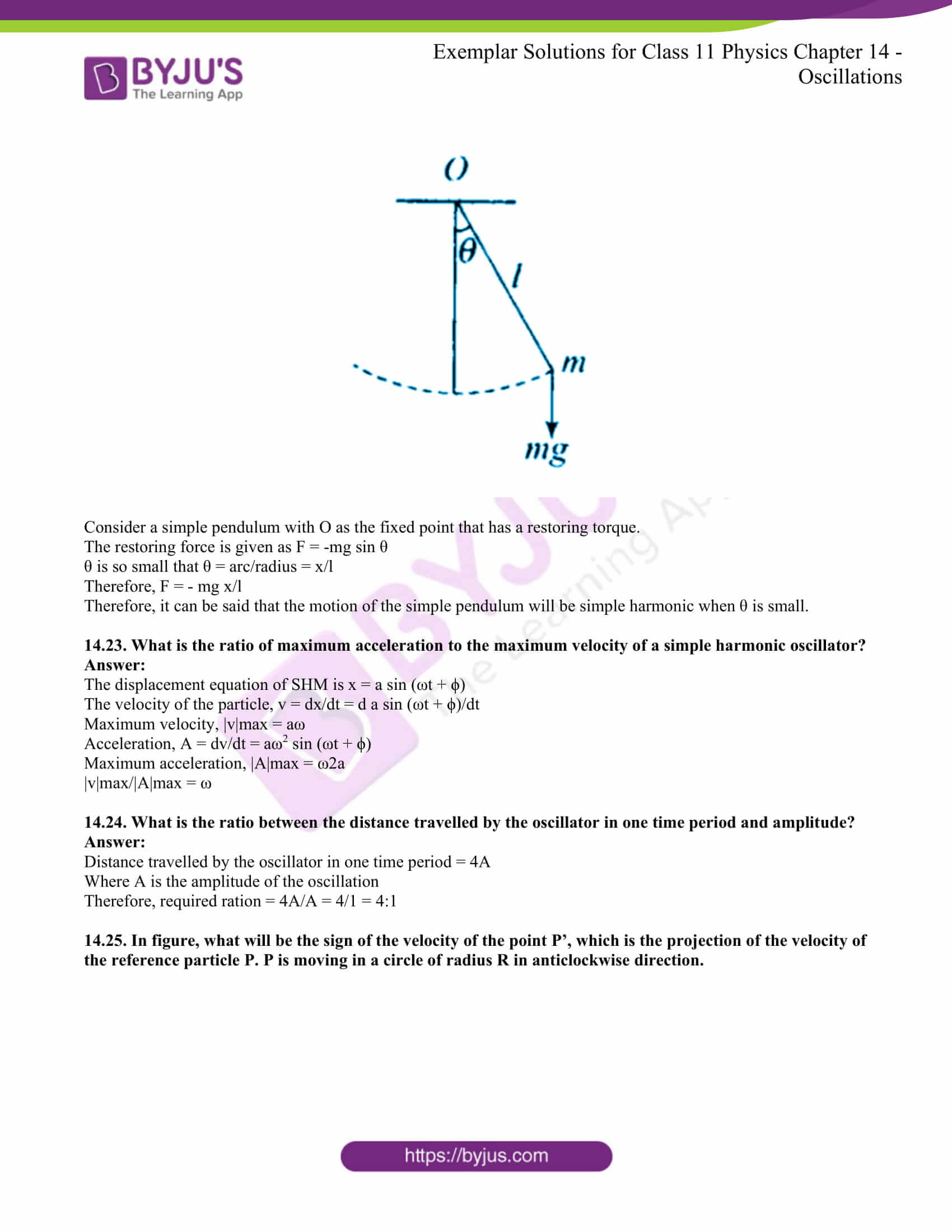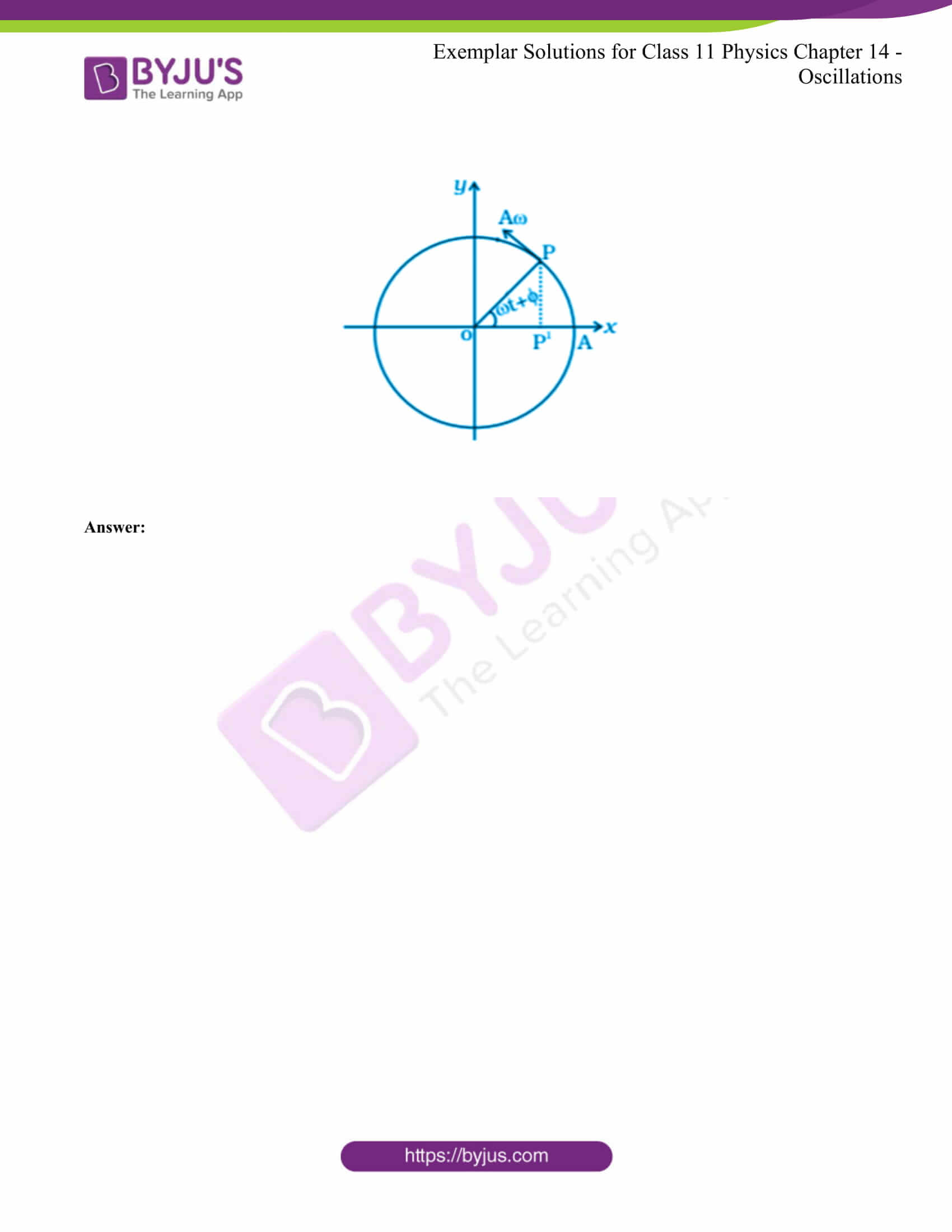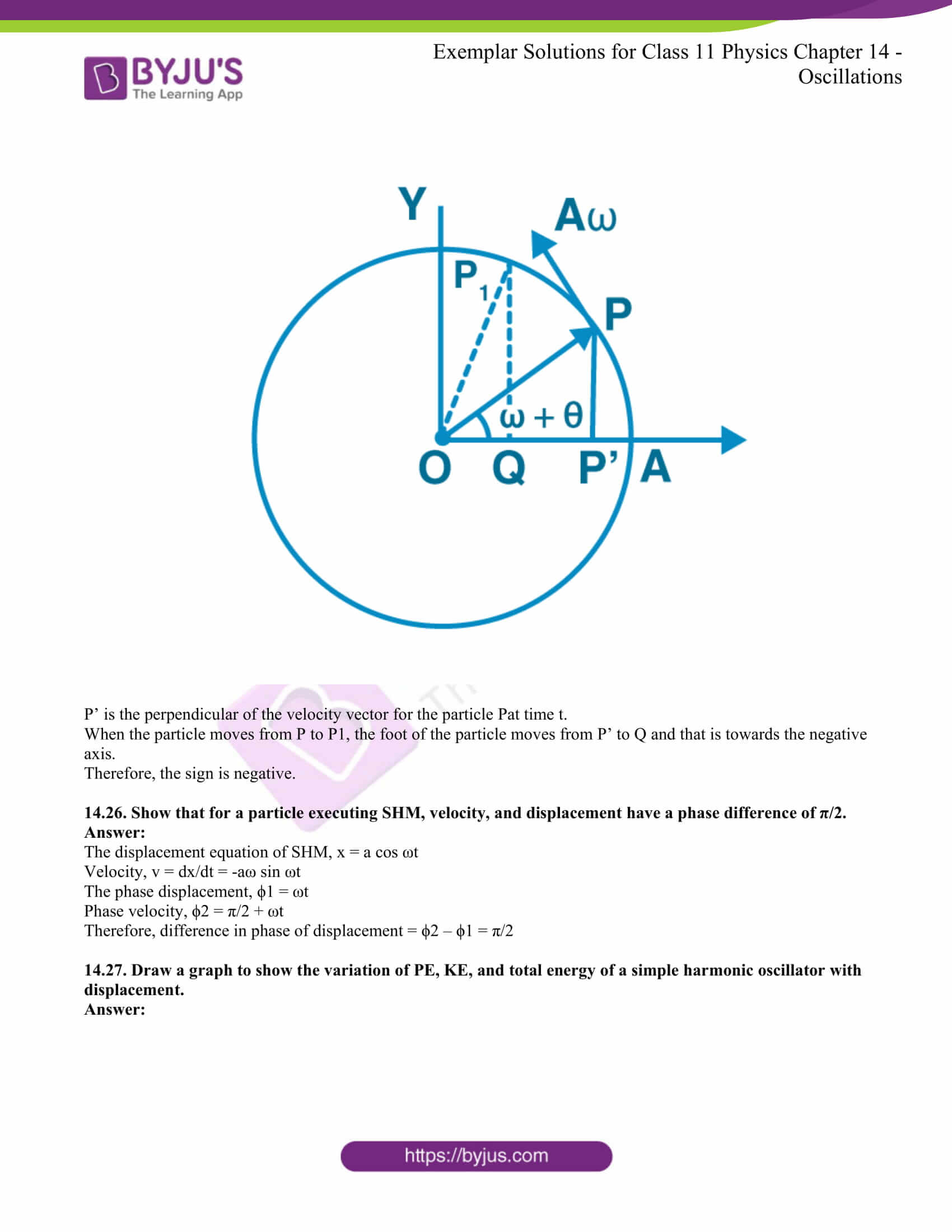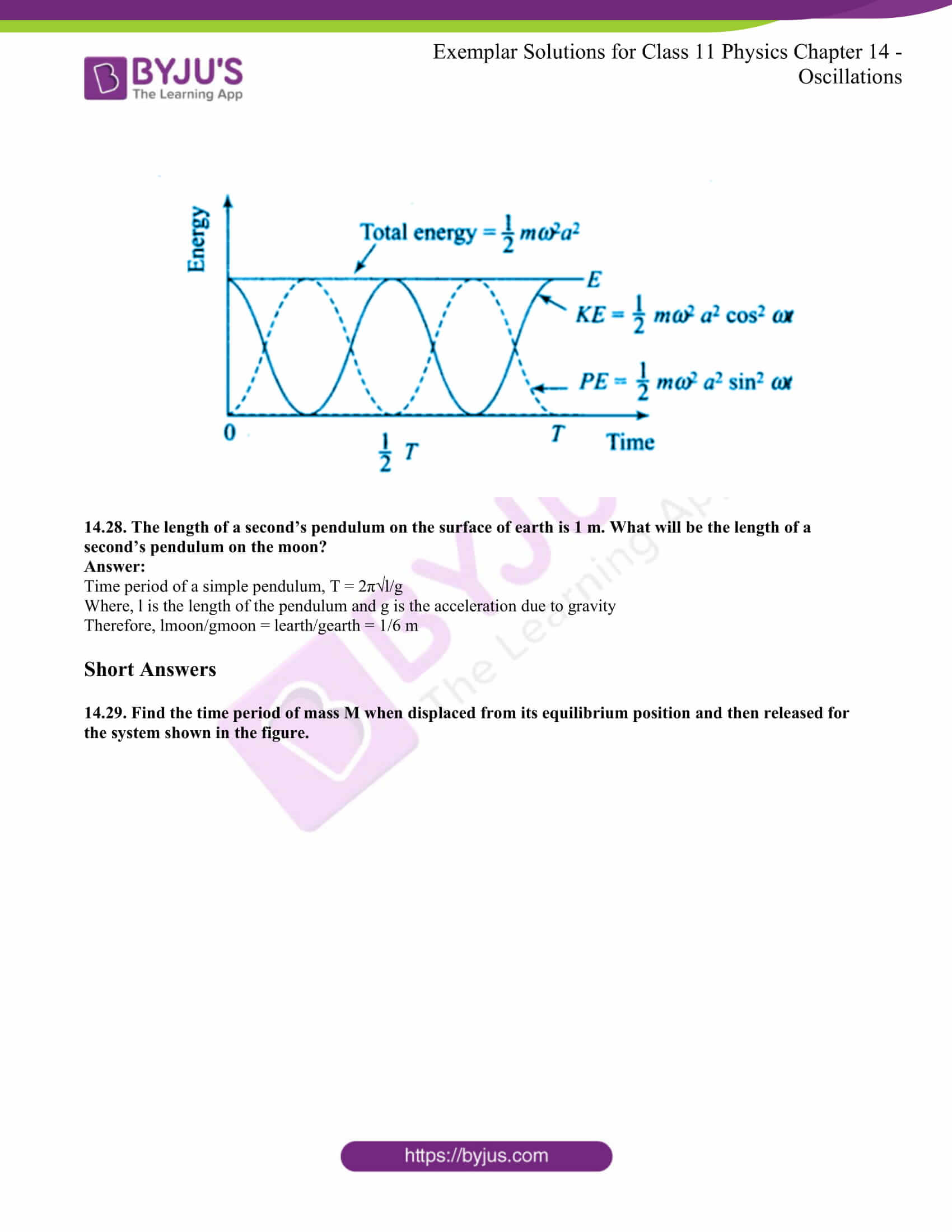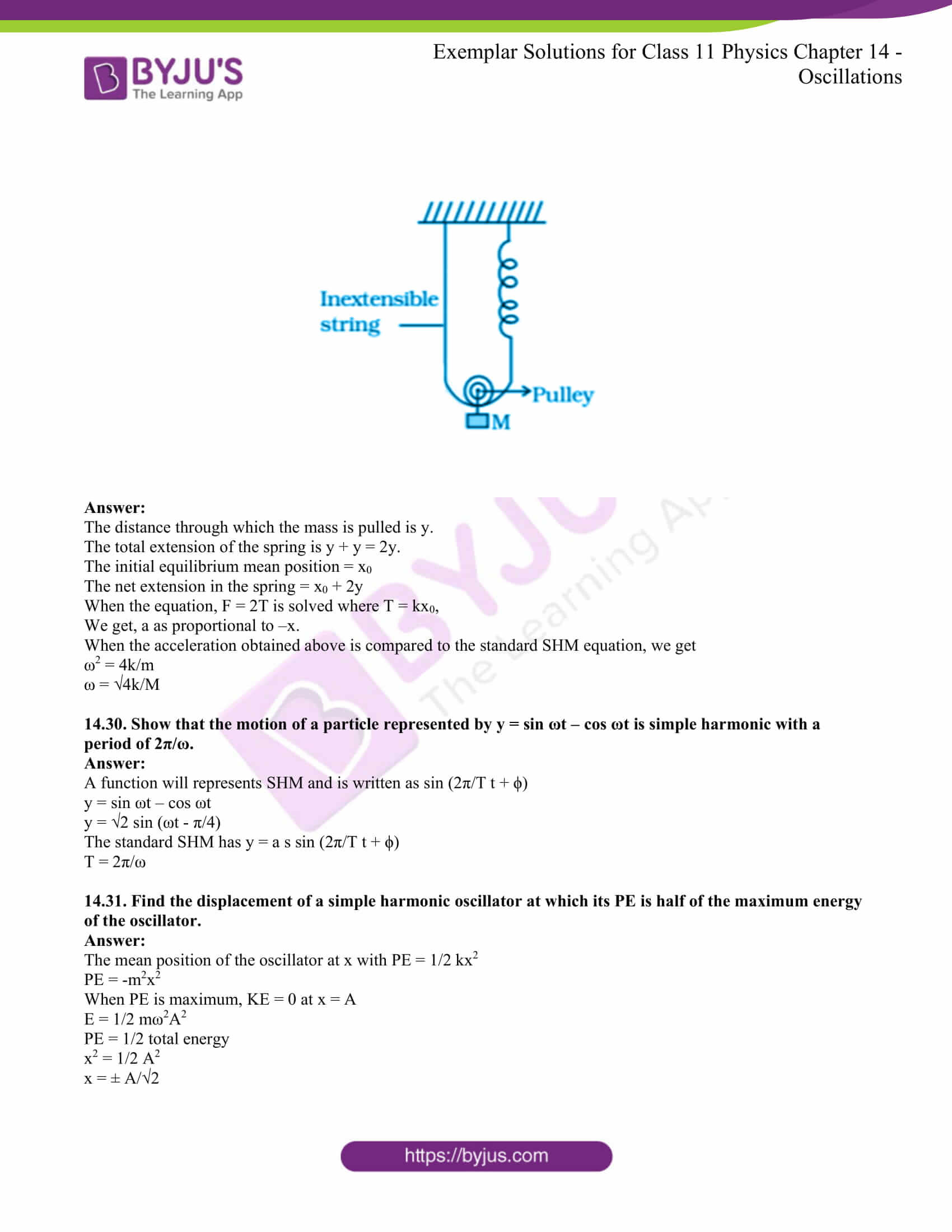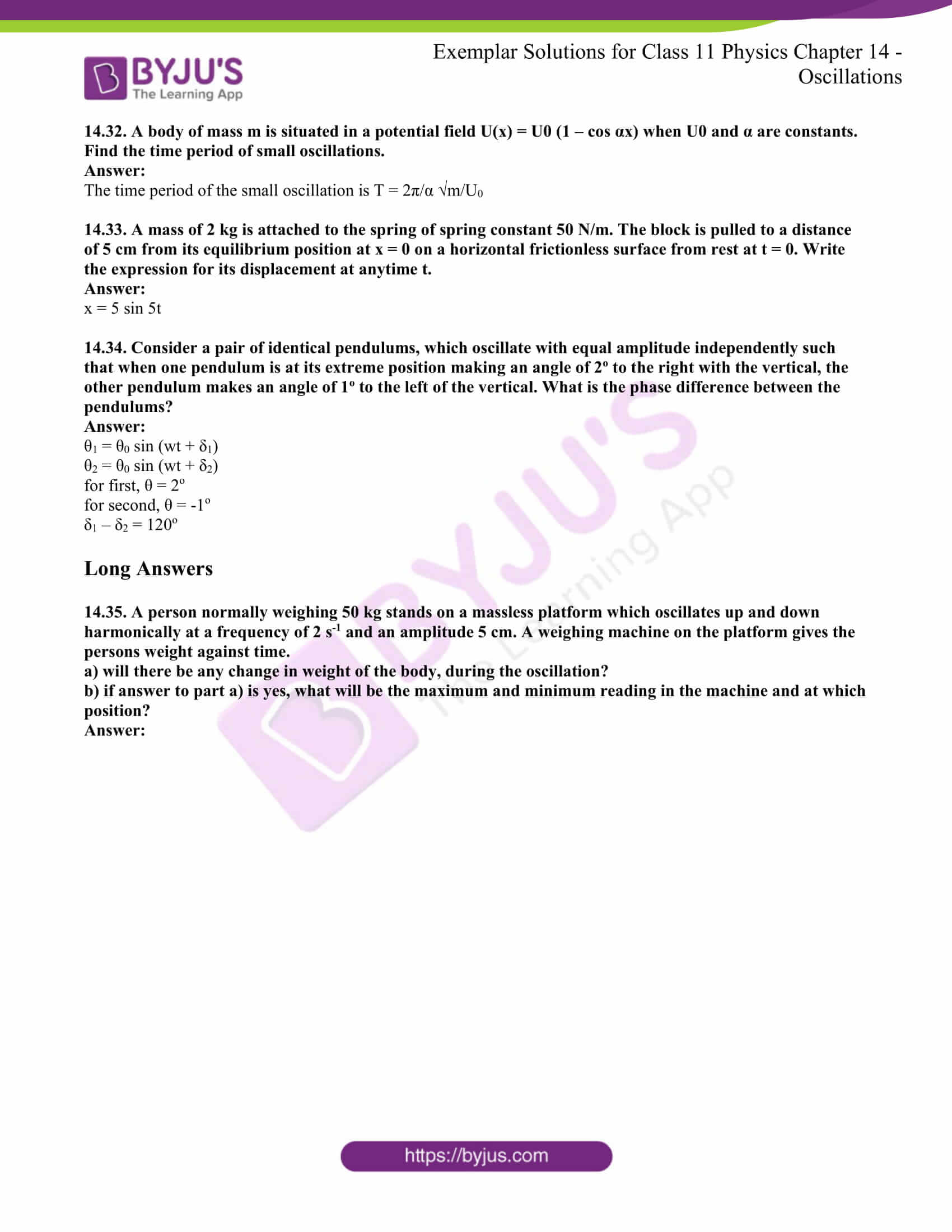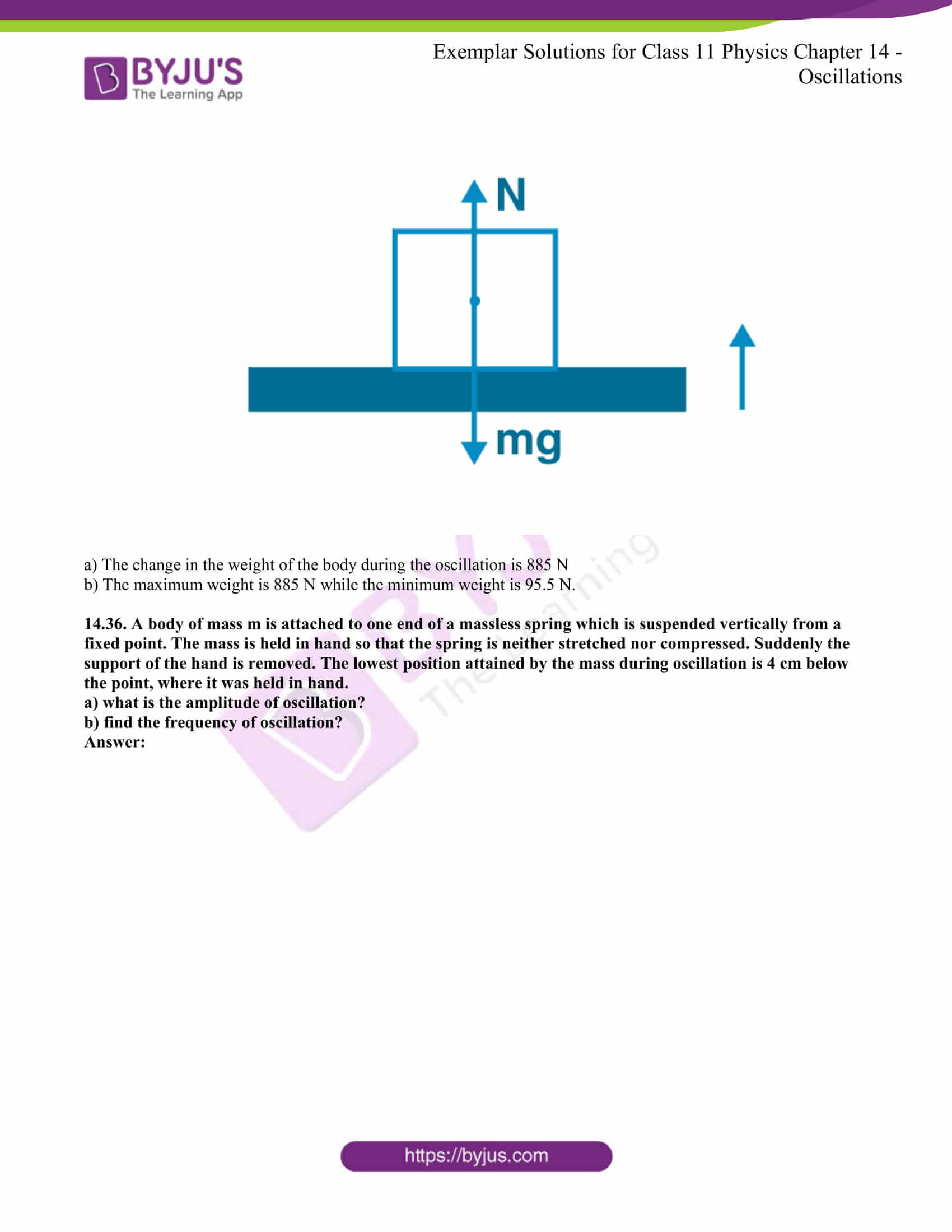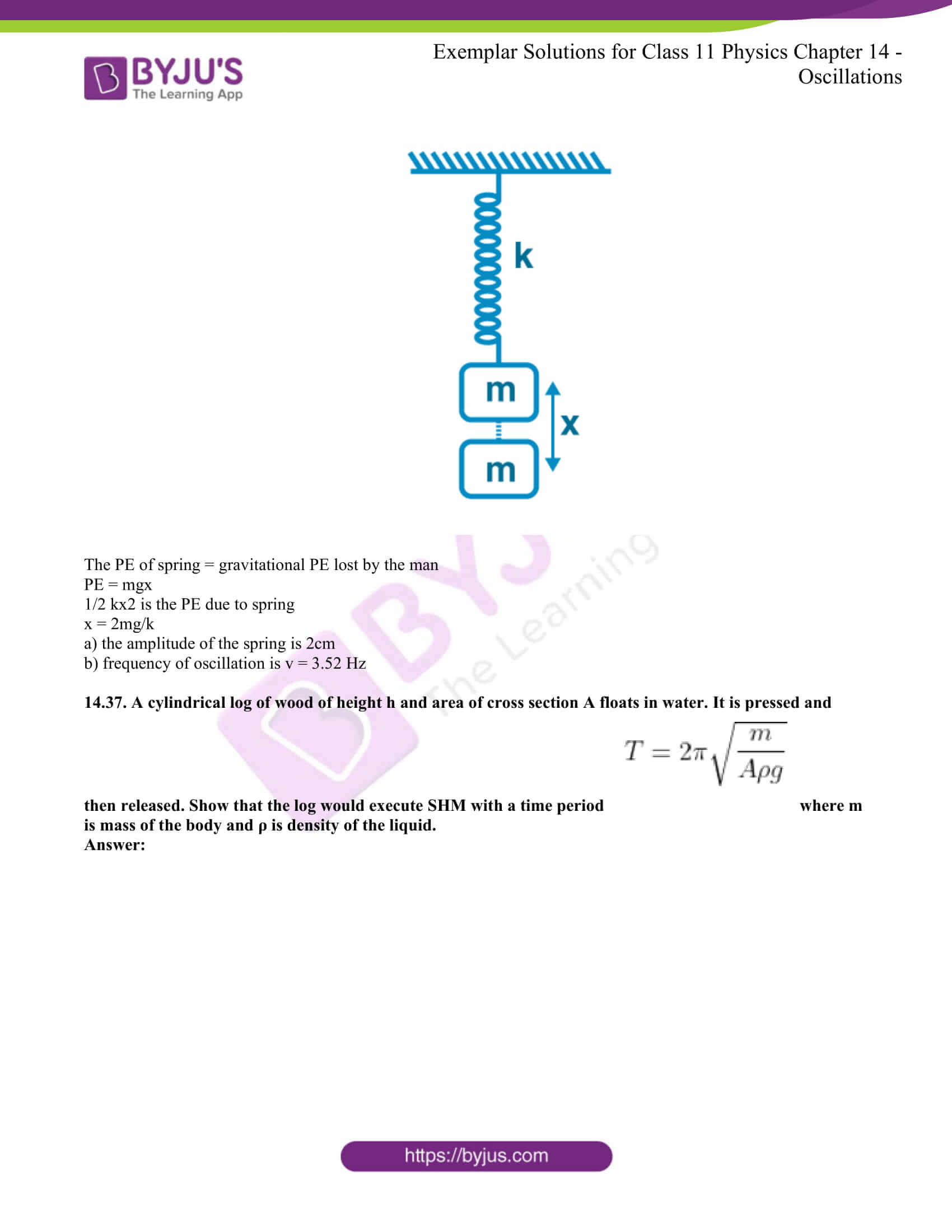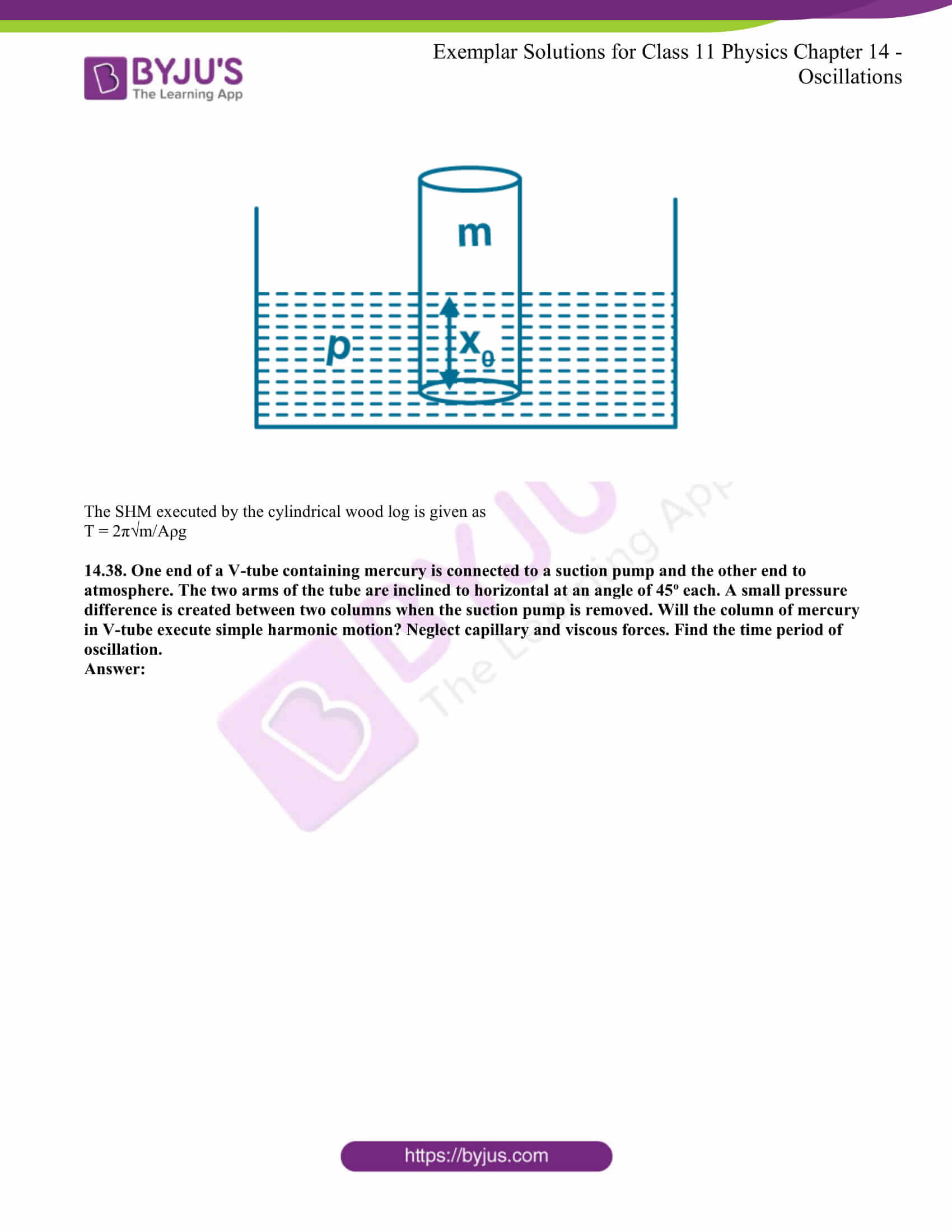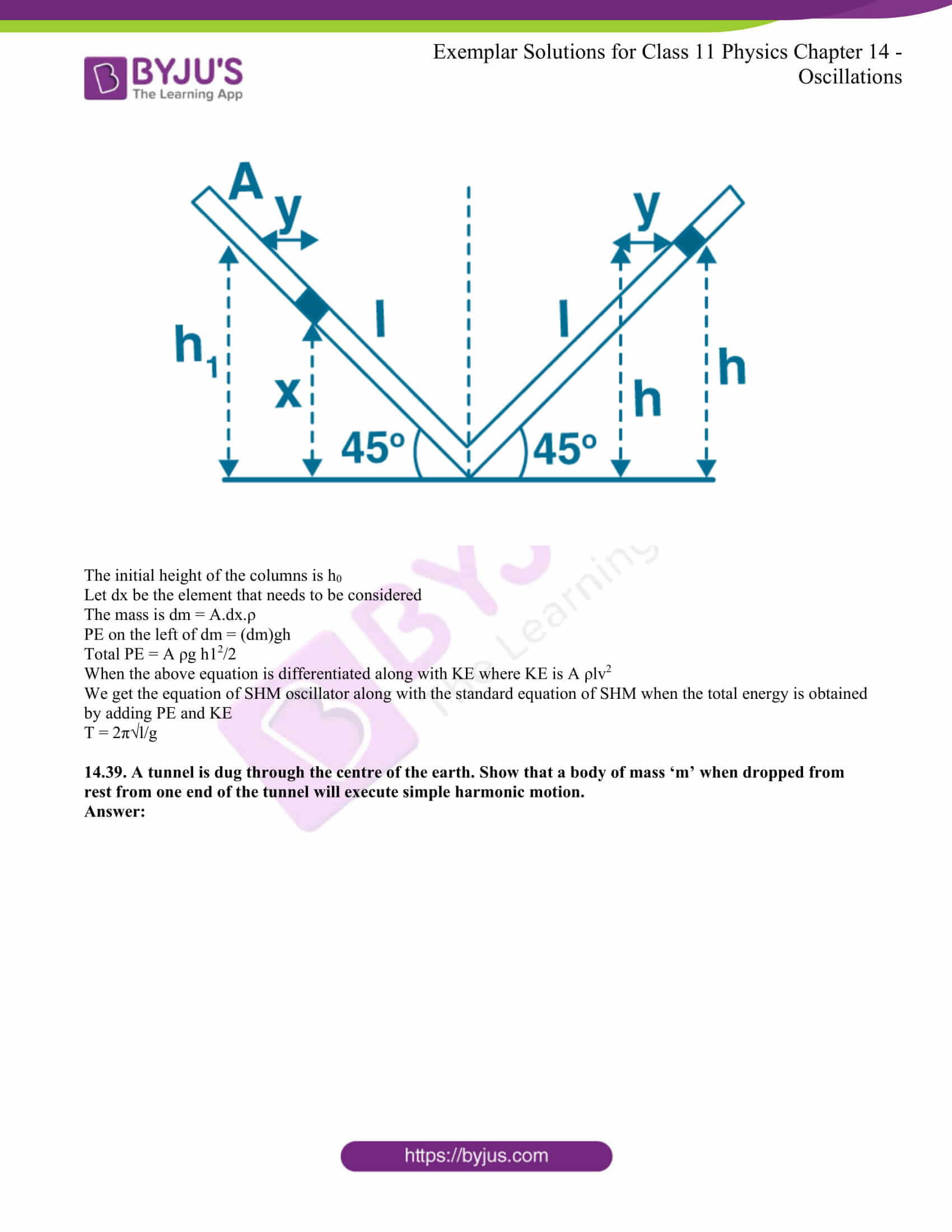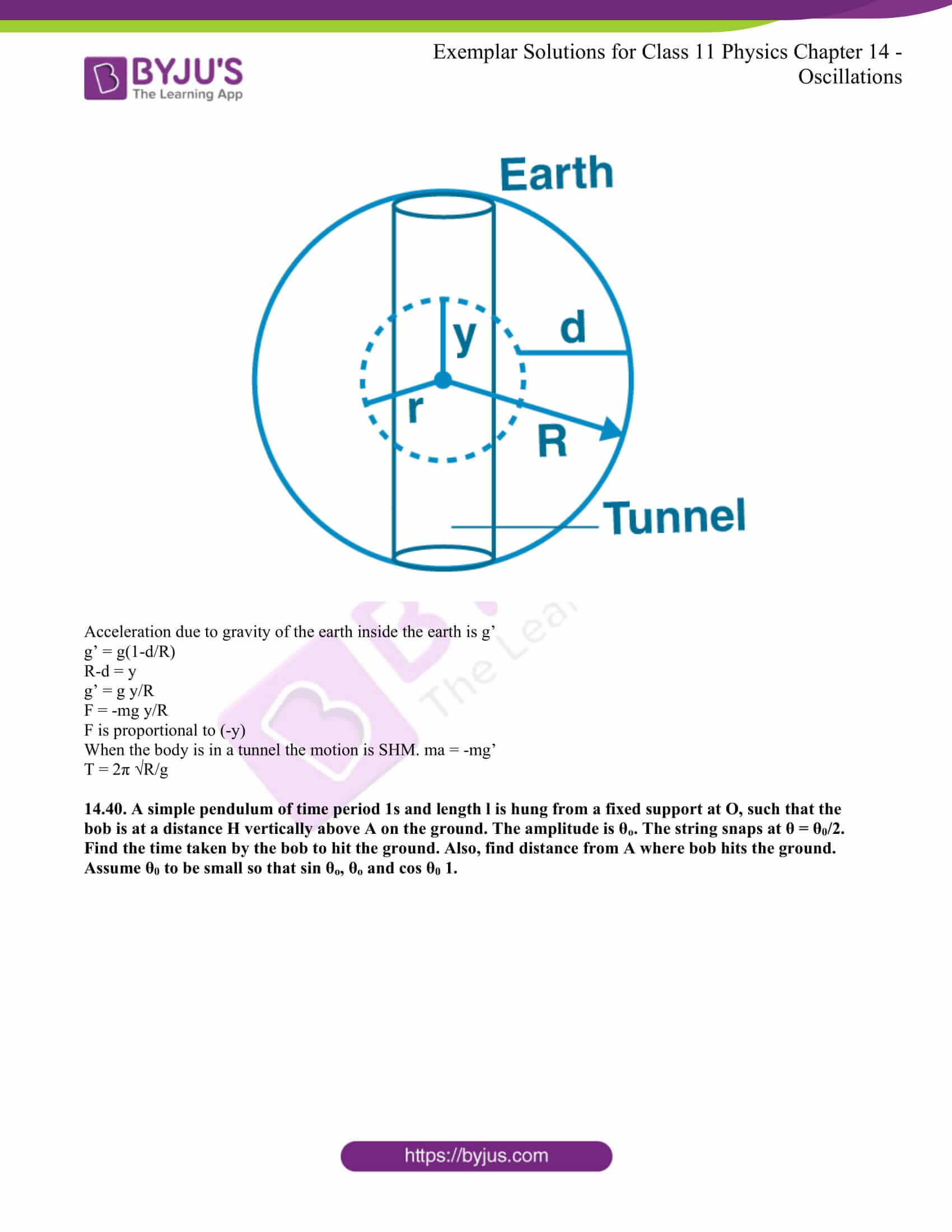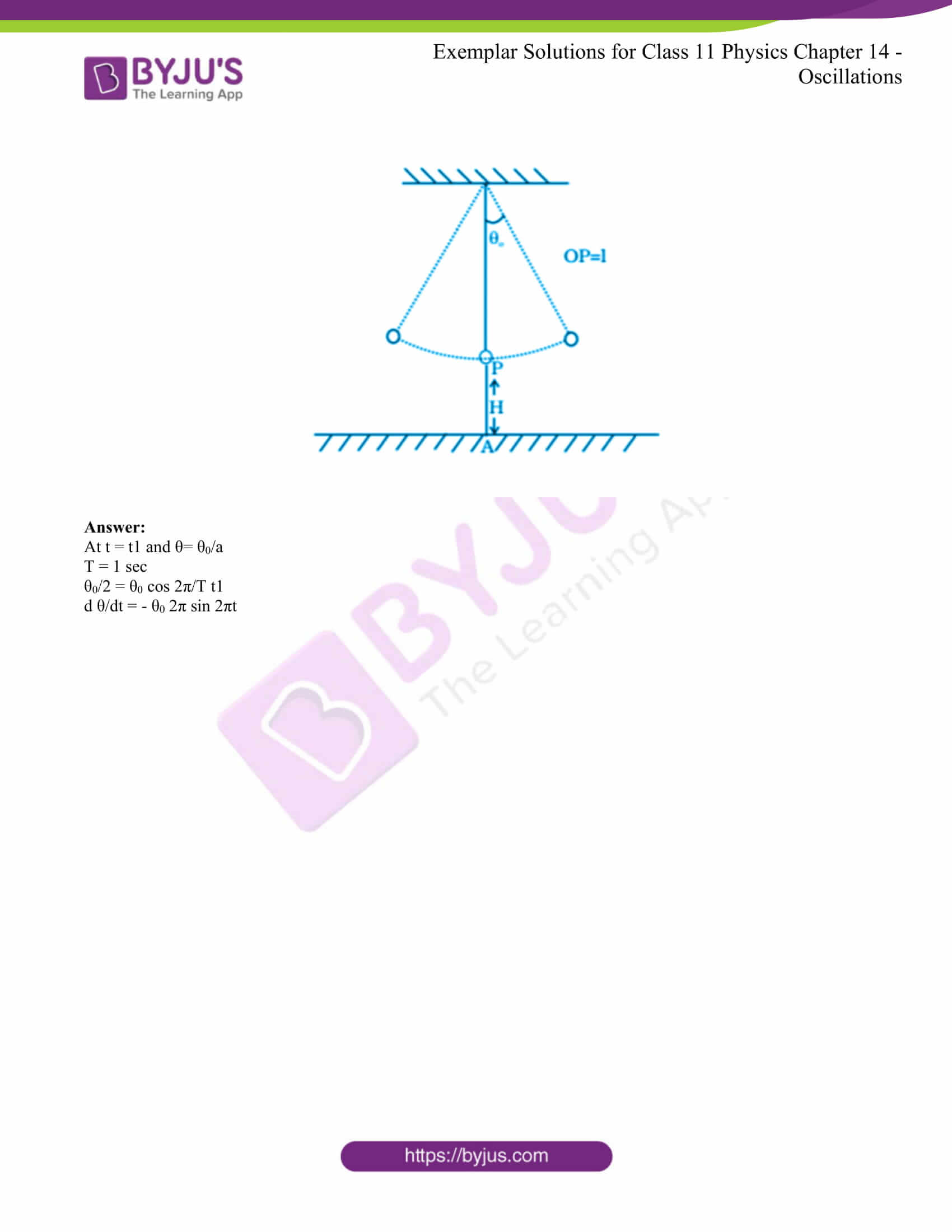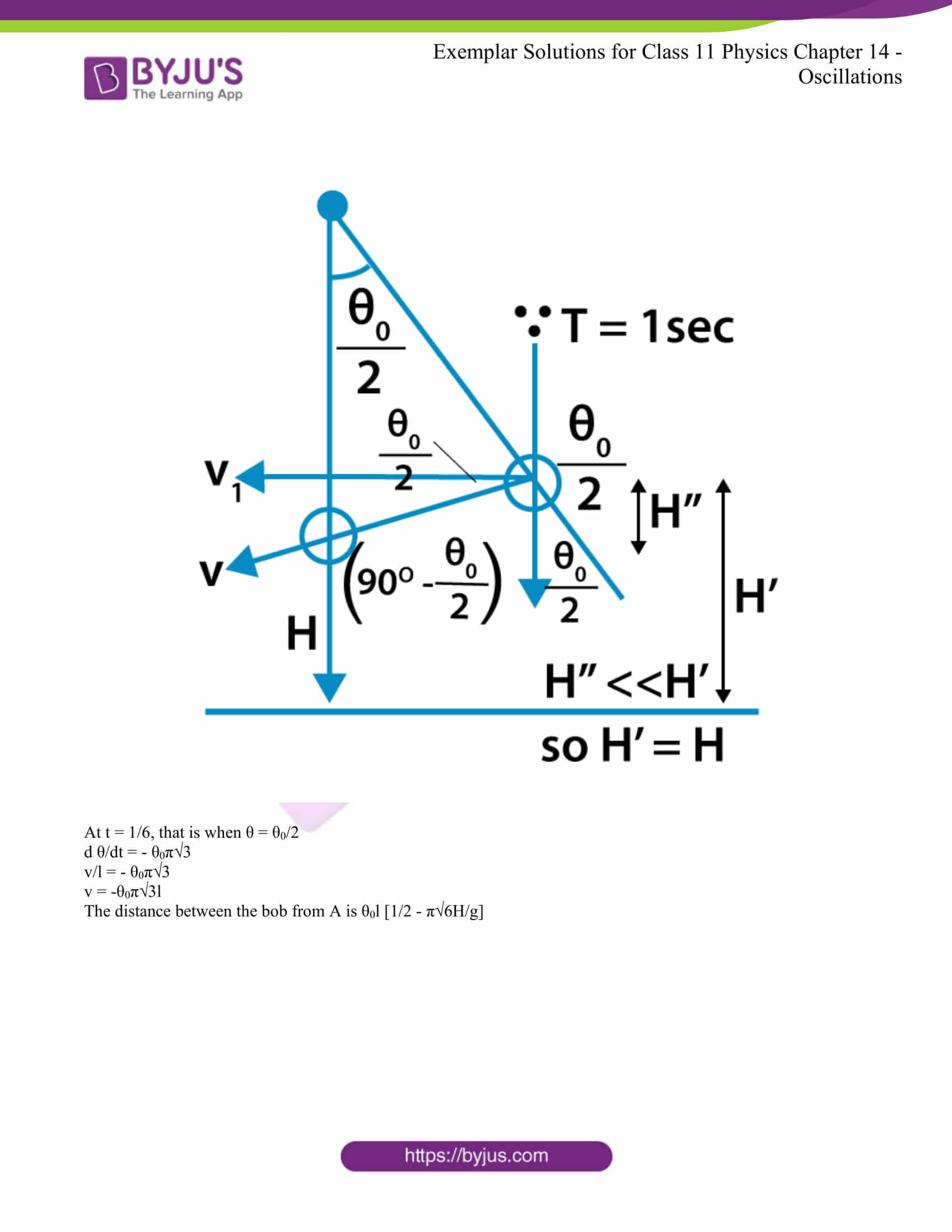### Multiple Choice Questions I

14.1. The displacement of a particle is represented by the equation y = 3 cos(π/4 – 2ωt). The motion of the particle is

a) simple harmonic with period 2p/w

b) simple harmonic with period π/ω

c) periodic but not simple harmonic

d) non-periodic

The correct answer is b) simple harmonic with period π/ω

14.2. The displacement of a particle is represented by the equation y = sin3 ωt. The motion is

a) non-periodic

b) periodic but not simple harmonic

c) simple harmonic with period 2π/ω

d) simple harmonic with period π/ω

The correct answer is c) simple harmonic with period 2π/ω

14.3. The relation between acceleration and displacement of four particles are given below:

a) ax = +2x

b) ax = +2x2

c) ax = -2x2

d) ax = -2x

Which one of the particles is executing simple harmonic motion?

The correct answer is d) ax = -2x

14.4. Motion of an oscillating liquid column in a U-tube is

a) periodic but not simple harmonic

b) non-periodic

c) simple harmonic and time period is independent of the density of the liquid

d) simple harmonic and time period is directly proportional to the density of the liquid

The correct answer is c) simple harmonic and time period is independent of the density of the liquid

14.5. A particle is acted simultaneously by mutually perpendicular simple harmonic motions x = a cos ωt and y = a sin ωt. The trajectory of motion of the particle will be

a) an ellipse

b) a parabola

c) a circle

d) a straight line

The correct answer is c) a circle

14.6. The displacement of a particle varies with time according to the relation y = a sin ωt + b cos ωt

a) the motion is oscillatory but not SHM

b) the motion is SHM with amplitude a + b

c) the motion is SHM with amplitude a2 + b2

d) the motion is SHM with amplitude √a2 + b2

The correct answer is d) the motion is SHM with amplitude √a2 + b2

14.7. Four pendulums A, B, C, and D are suspended from the same elastic support as shown in the figure. A and C are of the same length, while B is smaller than A and D is larger than A. If A is given a transverse displacement,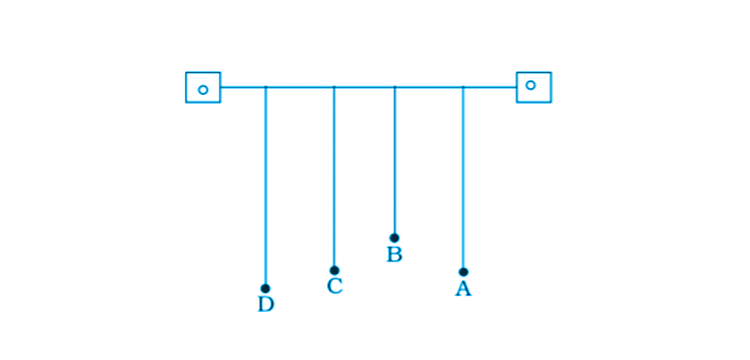a) D will vibrate with maximum amplitude

b) C will vibrate with maximum amplitude

c) B will vibrate with maximum amplitude

d) all the four will oscillate with equal amplitude

The correct answer b) C will vibrate with maximum amplitude

14.8. Figure shows the circular motion of a particle. The radius of the circle, the period, sense of revolution, and the initial position are indicated on the figure. The simple harmonic motion of the x-projection of the radius vector of the rotating particle P is

a) x(t) = B sin (2πt/30)

b) x(t) = B cos (πt/15)

c) x(t) = B sin (πt/15 + π/2)

d) x(t) = B cos (πt/15 + π/2)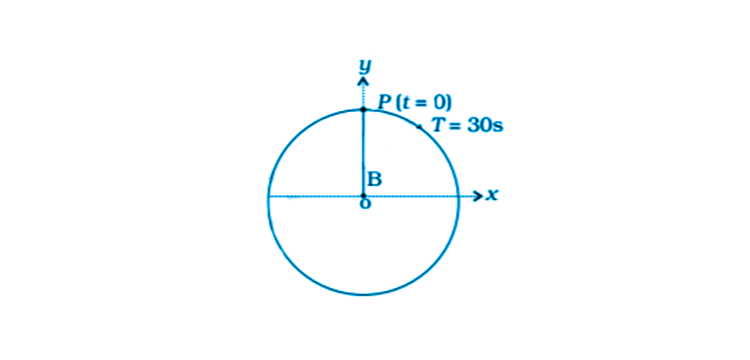The correct answer a) x(t) = B sin (2πt/30)

14.9. The equation of motion of a particle is x = a cos (αt)2. The motion is

a) periodic but not oscillatory

b) periodic and oscillatory

c) oscillatory but not periodic

d) neither periodic nor oscillatory

The correct answer c) oscillator but not periodic

14.10. A particle executing SHM has a maximum speed of 30 cm/s and a maximum acceleration of 60 cm/s2. The period of oscillation is

a) πs

b) π/2 s

c) 2 πs

d) π/t s

14.11. When a mass m is connected individually to two springs S1 and S2, the oscillation frequencies are v1 and v2. If the same mass is attached to the two springs are shown in the figure, the oscillation frequency would be

a) v1 + v2

b)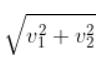c)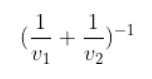d)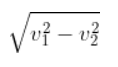### Multiple Choice Questions II

14.12. The rotation of the earth about its axis is

a) periodic motion

b) simple harmonic motion

c) periodic but not simple harmonic motion

d) non-periodic motion

a) periodic motion

c) periodic but not simple harmonic motion

14.13. The motion of a ball bearing inside a smooth curved bowl, when released from a point slightly above the lower point is

a) simple harmonic motion

b) non-periodic motion

c) periodic motion

d) periodic but not SHM

a) simple harmonic motion

b) non-periodic motion

14.14. Displacement versus time curve for a particle executing SHM is shown in the figure. Choose the correct statements.

a) phase of the oscillator is same at t = 0 s and t = 2 s

b) phase of the oscillator is the same at t = 2 s and t = 6 s

c) phase of the oscillator is the same at t = 1 s and t = 7 s

d) phase of the oscillator is the same at t = 1 s and t = 5 s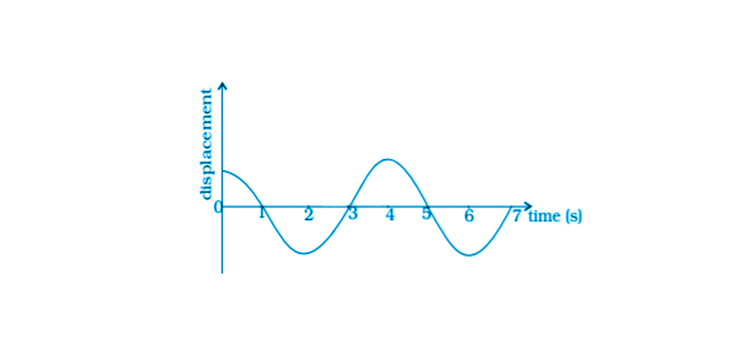b) phase of the oscillator is the same at t = 2 s and t = 6 s

d) phase of the oscillator is the same at t = 1 s and t = 5 s

14.15. Which of the following statements is/are true for a simple harmonic oscillator?

a) force acting is directly proportional to the displacement from the mean position and opposite to it

b) motion is periodic

c) acceleration of the oscillator is constant

d) the velocity is periodic

a) force acting is directly proportional to the displacement from the mean position and opposite to it

b) motion is periodic

d) the velocity is periodic

14.16. The displacement time graph of a particle executing SHM is shown in the figure. Which of the following statement is/are true?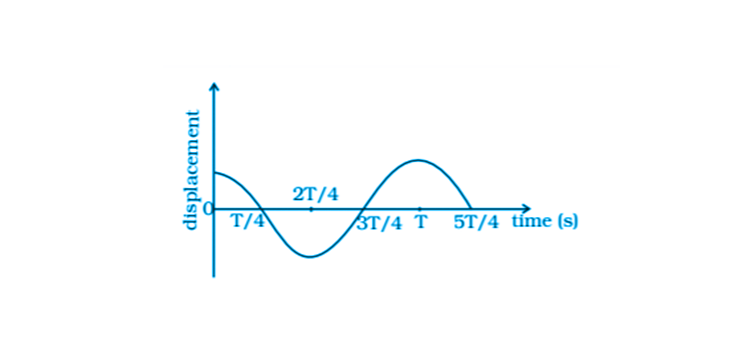a) the force is zero at t = 3T/4

b) the acceleration is maximum at t = 4T/4

c) the velocity is maximum at t = T/4

d) the PE is equal to KE of oscillation t = T/2

a) the force is zero at t = 3T/4

b) the acceleration is maximum at t = 4T/4

c) the velocity is maximum at t = T/4

14.17. A body is performing SHM. Then its

a) average total energy per cycle is equal to its maximum kinetic energy

b) average kinetic energy per cycle is equal to half of its maximum kinetic energy

c) mean velocity over a complete cycle is equal to 2/π times of its maximum velocity

d) root mean square velocity is 1/√2 times of its maximum velocity

a) average total energy per cycle is equal to its maximum kinetic energy

b) average kinetic energy per cycle is equal to half of its maximum kinetic energy

d) root mean square velocity is 1/√2 times of its maximum velocity

14.18. A particle is in linear simple harmonic motion between two points A and B, 10 cm apart. Taken the direction from A to B as the +ve direction and choose the correct statements.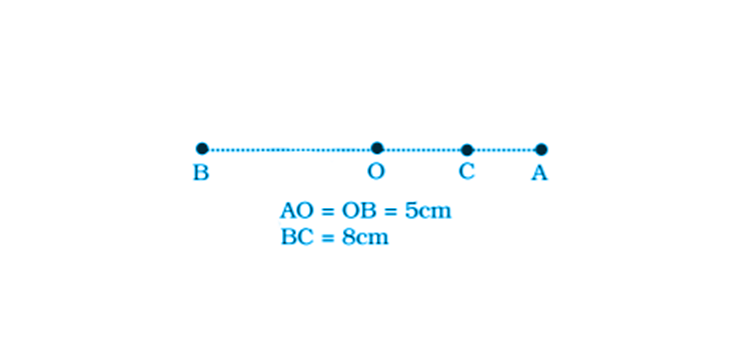a) the sign of velocity, acceleration, and force on the particle when it is 3 cm away from A going towards B are positive

b) the sign of velocity of the particle at C going towards O is negative

c) the sign of velocity, acceleration, and force on the particle when it is 4 cm away from B going towards A are negative

d) the sign of acceleration and force on the particle when it is at point B is negative

a) the sign of velocity, acceleration, and force on the particle when it is 3 km away from A going towards B are positive

c) the sign of velocity, acceleration, and force on the particle when it is 4 cm away from B going towards A are negative

d) the sign of acceleration and force on the particle when it is at point B is negative

14.19. Displacement versus time curve for a particle executing SHM is shown. Identify the points marked at which

i) velocity of the oscillator is zero

ii) speed of the oscillator is maximum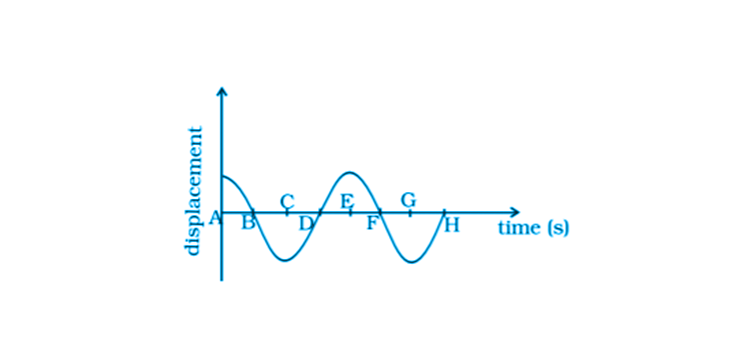i) When the points A, C, E, and G lie at their extreme positions, the velocity of the oscillator is zero.

ii) When the points B, D, F, and H lie at their mean positions, the speed of the oscillator is maximum.

14.20. Two identical springs of spring constant K are attached to a block of mass m and to fixed supports as shown in the figure. When the mass is displaced from equilibrium position by a distance x towards the right, find the restoring force.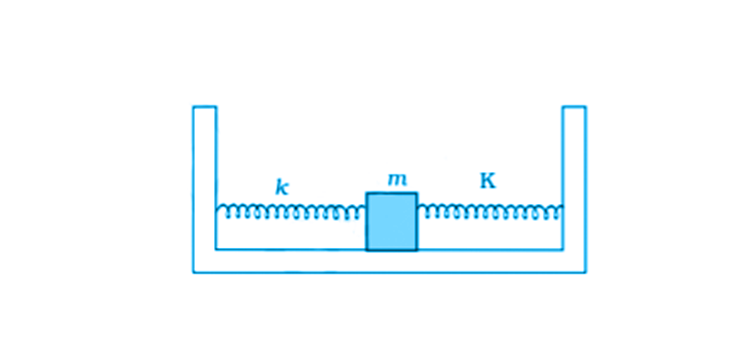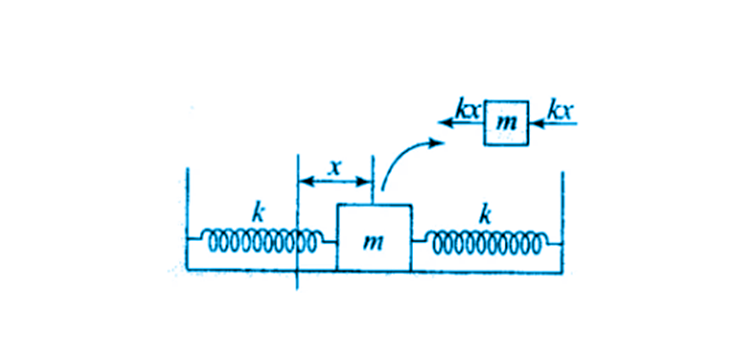Given,

F1 = -kx for the left spring

F2 = -kx for the right spring

Restoring force, F = F1 + F2 = -2kx

Therefore, when the spring is towards left, F = 2kx

14.21. What are the two basic characteristics of a simple harmonic motion?

Following are the two basic characteristics of an SHM:

a) acceleration and displacement are directly proportional to each other

b) the direction of acceleration is towards the mean position while the direction of displacement is towards the opposite side of the mean position

14.22. When will the motion of a simple pendulum be simple harmonic?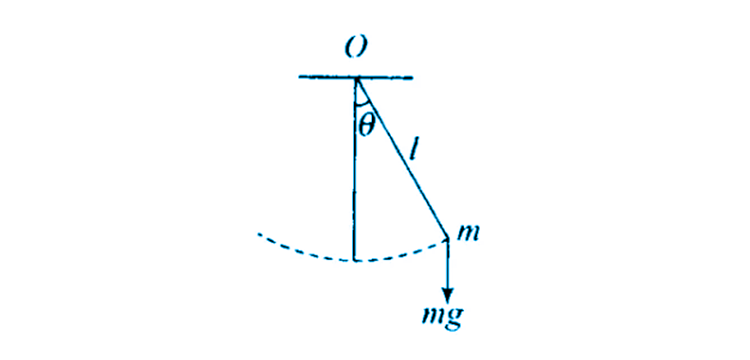Consider a simple pendulum with O as the fixed point that has a restoring torque.

The restoring force is given as F = -mg sin θ

θ is so small that θ = arc/radius = x/l

Therefore, F = – mg x/l

Therefore, it can be said that the motion of the simple pendulum will be simple harmonic when θ is small.

14.23. What is the ratio of maximum acceleration to the maximum velocity of a simple harmonic oscillator?

The displacement equation of SHM is x = a sin (ꞷt + ϕ)

The velocity of the particle, v = dx/dt = d a sin (ꞷt + ϕ)/dt

Maximum velocity, |v|max = aꞷ

Acceleration, A = dv/dt = aꞷ2 sin (ꞷt + ϕ)

Maximum acceleration, |A|max = ꞷ2a

|v|max/|A|max = ꞷ

14.24. What is the ratio between the distance travelled by the oscillator in one time period and amplitude?

Distance travelled by the oscillator in one time period = 4A

Where A is the amplitude of the oscillation

Therefore, required ration = 4A/A = 4/1 = 4:1

14.25. In figure, what will be the sign of the velocity of the point P’, which is the projection of the velocity of the reference particle P. P is moving in a circle of radius R in the anticlockwise direction.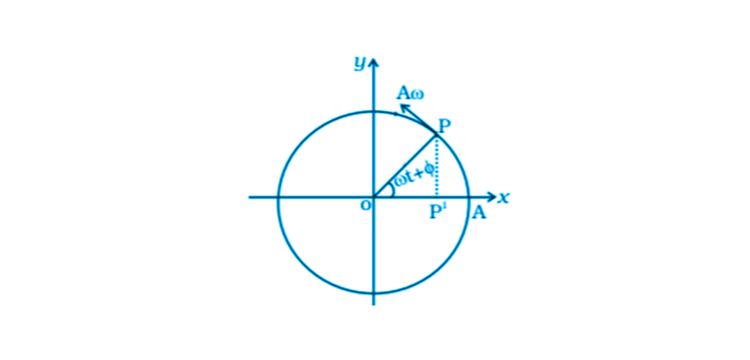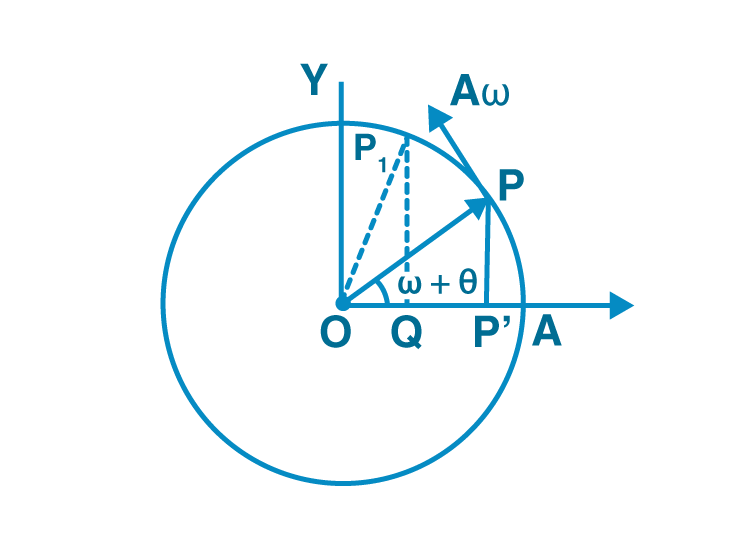P’ is the perpendicular of the velocity vector for the particle Pat time t.

When the particle moves from P to P1, the foot of the particle moves from P’ to Q and that is towards the negative axis.

Therefore, the sign is negative.

14.26. Show that for a particle executing SHM, velocity, and displacement have a phase difference of π/2.

The displacement equation of SHM, x = a cos ꞷt

Velocity, v = dx/dt = -aꞷ sin ꞷt

The phase displacement, ϕ1 = ꞷt

Phase velocity, ϕ2 = π/2 + ꞷt

Therefore, difference in phase of displacement = ϕ2 – ϕ1 = π/2

14.27. Draw a graph to show the variation of PE, KE, and total energy of a simple harmonic oscillator with displacement.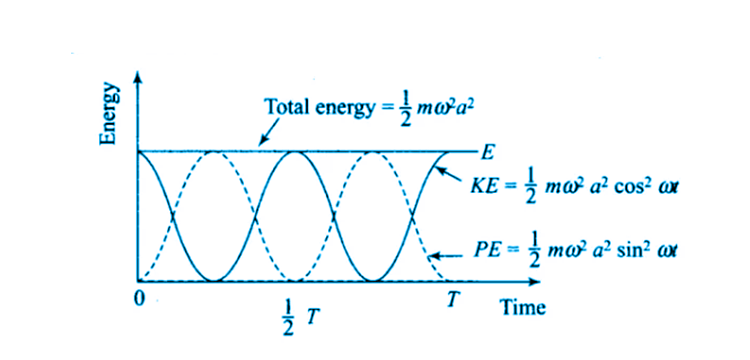14.28. The length of a second’s pendulum on the surface of the earth is 1 m. What will be the length of a second’s pendulum on the moon?

The time period of a simple pendulum, T = 2π√l/g

Where l is the length of the pendulum and g is the acceleration due to gravity

Therefore, lmoon/gmoon = learth/gearth = 1/6 m

14.29. Find the time period of mass M when displaced from its equilibrium position and then released for the system shown in the figure.The distance through which the mass is pulled is y.

The total extension of the spring is y + y = 2y.

The initial equilibrium mean position = x0

The net extension in the spring = x0 + 2y

When the equation, F = 2T is solved where T = kx0,

We get, a as proportional to –x.

When the acceleration obtained above is compared to the standard SHM equation, we get

2 = 4k/m

ꞷ = √4k/M

14.30. Show that the motion of a particle represented by y = sin ωt – cos ωt is simple harmonic with a period of 2π/ω.

A function will represent SHM and is written as sin (2π/T t + ϕ)

y = sin ωt – cos ωt

y = √2 sin (ωt – π/4)

The standard SHM has y = a s sin (2π/T t + ϕ)

T = 2π/ω

14.31. Find the displacement of a simple harmonic oscillator at which its PE is half of the maximum energy of the oscillator.

The mean position of the oscillator at x with PE = 1/2 kx2

PE = -m2x2

When PE is maximum, KE = 0 at x = A

E = 1/2 mω2A2

PE = 1/2 total energy

x2 = 1/2 A2

x = ± A/√2

14.32. A body of mass m is situated in a potential field U(x) = U0 (1 – cos αx) when U0 and α are constants. Find the time period of small oscillations.

The time period of the small oscillation is T = 2π/α √m/U0

14.33. A mass of 2 kg is attached to the spring of spring constant 50 N/m. The block is pulled to a distance of 5 cm from its equilibrium position at x = 0 on a horizontal frictionless surface from rest at t = 0. Write the expression for its displacement at anytime t.

x = 5 sin 5t

14.34. Consider a pair of identical pendulums, which oscillate with equal amplitude independently such that when one pendulum is at its extreme position making an angle of 2o to the right with the vertical, the other pendulum makes an angle of 1o to the left of the vertical. What is the phase difference between the pendulums?

θ1 = θ0 sin (wt + δ1)

θ2 = θ0 sin (wt + δ2)

for first, θ = 2o

for second, θ = -1o

δ1 – δ2 = 120o

14.35. A person normally weighing 50 kg stands on a massless platform which oscillates up and down harmonically at a frequency of 2 s-1 and an amplitude 5 cm. A weighing machine on the platform gives the persons weight against time.

a) will there be any change in weight of the body, during the oscillation?

b) if the answer to part a) is yes, what will be the maximum and minimum reading in the machine and at which position?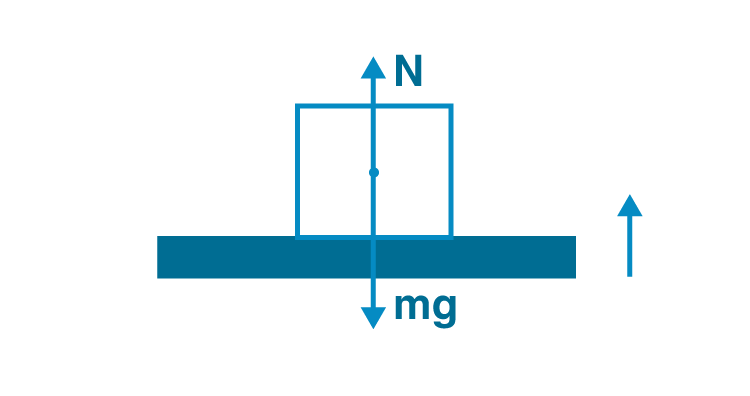a) The change in the weight of the body during the oscillation is 885 N

b) The maximum weight is 885 N while the minimum weight is 95.5 N.

14.36. A body of mass m is attached to one end of a massless spring which is suspended vertically from a fixed point. The mass is held in hand so that the spring is neither stretched nor compressed. Suddenly the support of the hand is removed. The lowest position attained by the mass during oscillation is 4 cm below the point, where it was held in hand.

a) what is the amplitude of oscillation?

b) find the frequency of oscillation?The PE of spring = gravitational PE lost by the man

PE = mgx

1/2 kx2 is the PE due to spring

x = 2mg/k

a) the amplitude of the spring is 2cm

b) frequency of oscillation is v = 3.52 Hz

14.37. A cylindrical log of wood of height h and area of cross-section A floats in water. It is pressed and then released. Show that the log would execute SHM with a time period where m is mass of the body and ρ is the density of the liquid.The SHM executed by the cylindrical wood log is given as

T = 2π√m/Aρg

14.38. One end of a V-tube containing mercury is connected to a suction pump and the other end to the atmosphere. The two arms of the tube are inclined to horizontal at an angle of 45o each. A small pressure difference is created between two columns when the suction pump is removed. Will the column of mercury in V-tube execute simple harmonic motion? Neglect capillary and viscous forces. Find the time period of oscillation.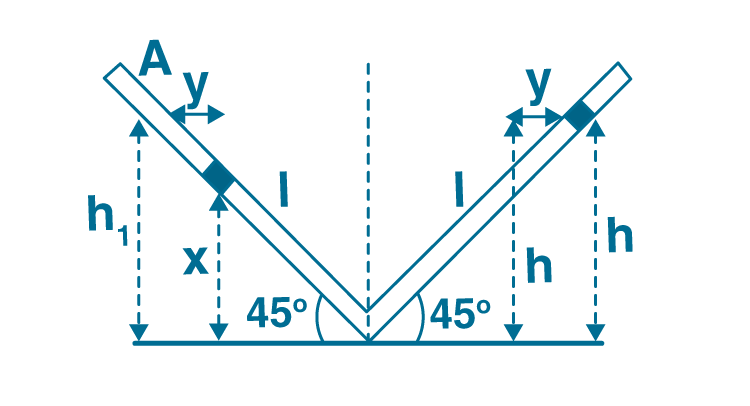The initial height of the columns is h0

Let dx be the element that needs to be considered

The mass is dm = A.dx.ρ

PE on the left of dm = (dm)gh

Total PE = A ρg h12/2

When the above equation is differentiated along with KE where KE is A ρlv2

We get the equation of SHM oscillator along with the standard equation of SHM when the total energy is obtained by adding PE and KE

T = 2π√l/g

14.39. A tunnel is dug through the centre of the earth. Show that a body of mass ‘m’ when dropped from rest from one end of the tunnel will execute simple harmonic motion.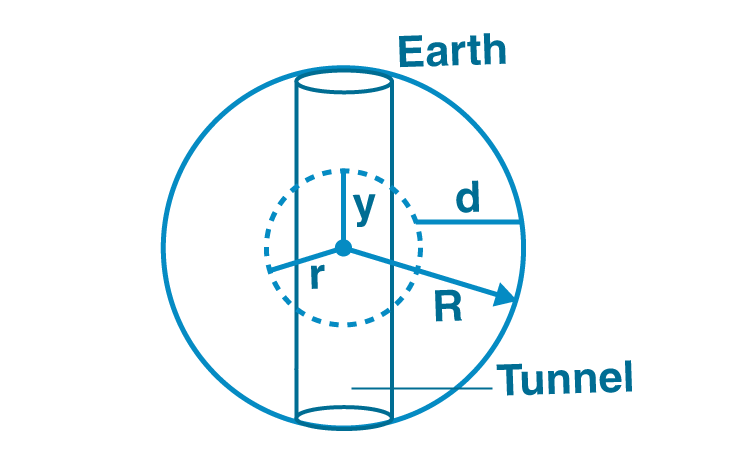Acceleration due to gravity of the earth inside the earth is g’

g’ = g(1-d/R)

R-d = y

g’ = g y/R

F = -mg y/R

F is proportional to (-y)

When the body is in a tunnel the motion is SHM. ma = -mg’

T = 2π √R/g

14.40. A simple pendulum of time period 1s and length l is hung from a fixed support at O, such that the bob is at a distance H vertically above A on the ground. The amplitude is θo. The string snaps at θ = θ0/2. Find the time taken by the bob to hit the ground. Also, find the distance from A where bob hits the ground. Assume θ0 to be small so that sin θo, θo and cos θ0 1.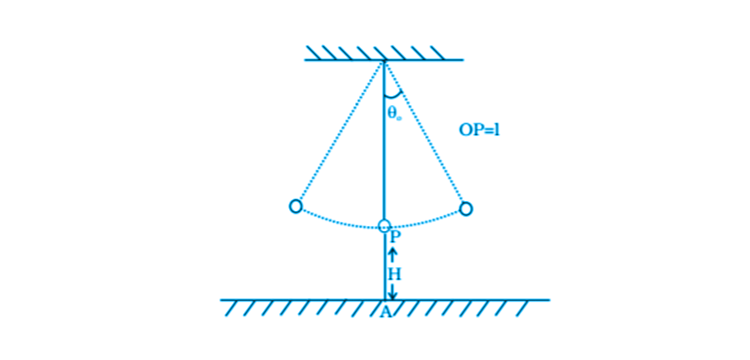At t = t1 and θ= θ0/a

T = 1 sec

θ0/2 = θ0 cos 2π/T t1

d θ/dt = – θ0 2π sin 2πt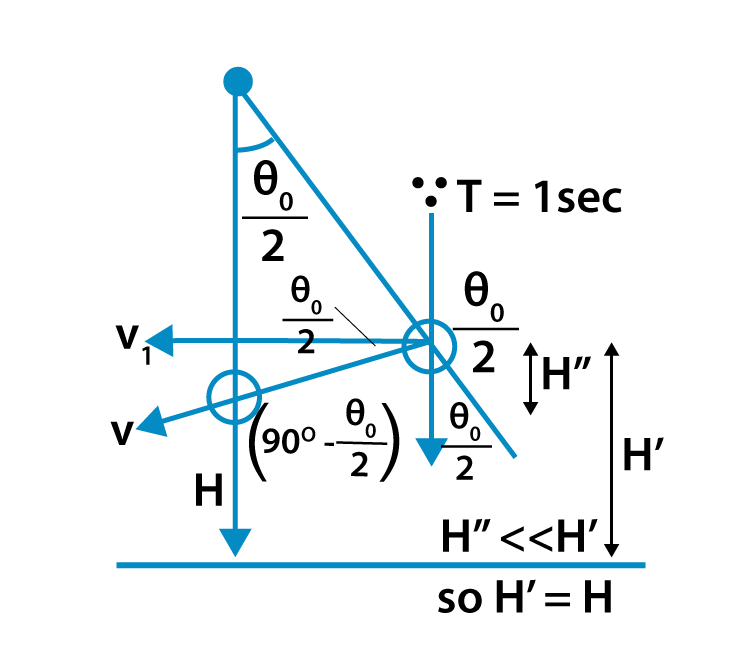At t = 1/6, that is when θ = θ0/2

d θ/dt = – θ0π√3

v/l = – θ0π√3

v = -θ0π√3l

The distance between the bob from A is θ0l [1/2 – π√6H/g]

Oscillation has many different uses and real-life applications with examples such as:

1. Tuning fork
2. Vibrating string
3. Torsional vibration
4. Oscillator

## Important topics of class 11 physics chapter 14 oscillations

1. Periodic and oscillatory motions
2. Simple harmonic motion
3. Simple harmonic motion and uniform circular motion
4. Velocity and acceleration in simple harmonic motion
5. Force law for simple harmonic motion
6. Energy in simple harmonic motion
7. Some systems executing SHM Ex
8. Damped simple harmonic motion
9. Forced oscillations and resonance.

### Why to Opt BYJU’s?

Visit BYJU’S to get updated materials to prepare well for your class 11 final exams and entrance examinations. Download and try BYJU’s – The Learning app for a more interactive and seamless learning experience with the help of videos which explains the concepts of class 11 and 12 Physics topics.